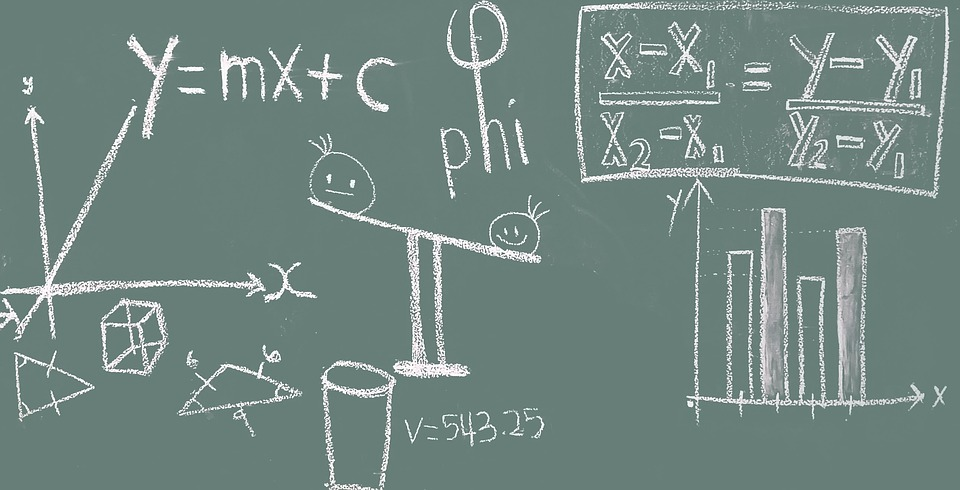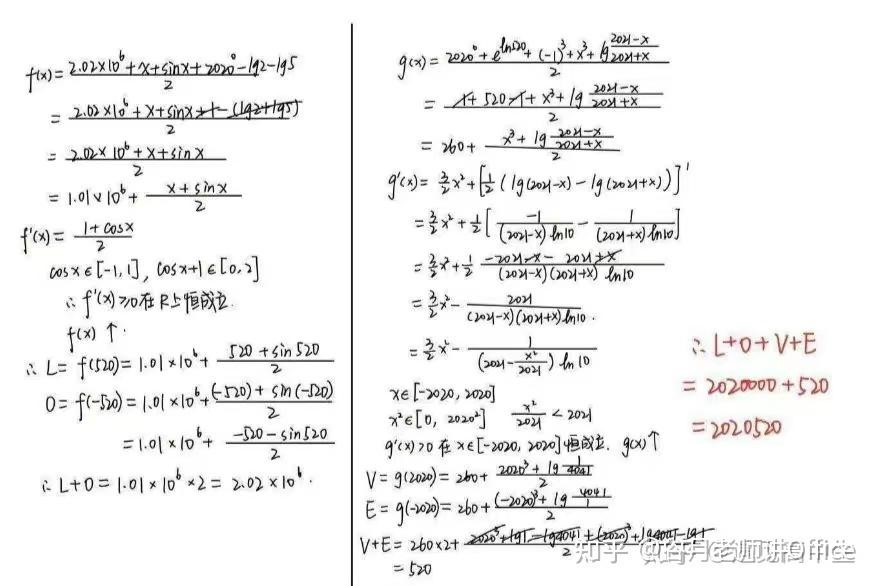# 让整体代换思想帮你解决数学疑难问题【例 1】（2020 天津中考）已知 $x+y=69$，求 $(x-34)^{99}+(y-35)^{99}=$ _____

【解析】此处如果用高中阶段的二项式定理展开会陷入无法合并化简的困境。

【例 2】（美国初中奥赛）已知 $x^2+x+1=0$，求 $x^{49}+x^{50}+x^{51}+x^{52}+x^{53}=$ _____

【解析】显然 $x^2+x+1=0$ 没有实数根，如果用求根公式求出复数根再代入待求式来运算，将会十分麻烦。

【例 3】（2023 北京中考）若 $x^2-y^2=16$$(x+y)^2=8$，求 $xy$ 的值。

【解析】已知条件是一个二元二次方程组，直接求解 $x$$y$ 相当麻烦。分析一下已知条件和待求结果，发现把题给条件中的完全平方式展开一下就会出现 $xy$。展开得到 $\left\{\begin{array}{l} x^2-y^2=16 &...(1) \\\\ x^2+2xy+y^2=8 &...(2) \end{array}\right.$

$(1)+(2)$ 得， $2x^2+2xy=24 \Rightarrow x(x+y)=12\quad ...(3)$

$(2)-(1)$ 得， $2y^2+2xy=-8 \Rightarrow y(x+y)=-4 \quad ...(4)$

$(3)\times(4)$ 得，$xy(x+y)^2=-48$，所以 $xy=\dfrac{-48}{(x+y)^2}=-6$

【反套路例题】（江苏丹阳中学高二月考）已知函数 $f(x)=\dfrac{2.02\times10^6+x+\sin x+2020^0-\lg 2-\lg 5}{2}$ 在区间 $[-520,520]$ 最大值和最小值分别为 $L,\ O$$g(x)=\dfrac{2020^0+e^{\ln 520}+(-1)^3+x^3+\lg{\dfrac{2021-x}{2021+x}}}{2}$$[-2020,2020]$ 上的最大值和最小值分别为 $V,\ E$，则 $L+O+V+E=$ ____________。

【解析】“我这么可爱，请给我钱 o(*^ω^*)o”https://blog.kukmoon.com/f461c1cf/

Kukmoon谷月

2023年10月13日